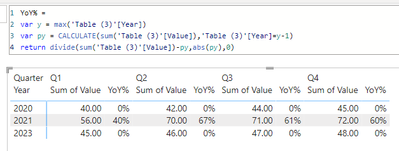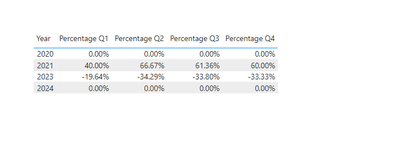cancel
Showing results for
Did you mean:Frequent Visitor

## Percentage calculation

Hi All,

Please find the below sanpshot.I have a data with this format.Based on that i want to calculate the percentage over each year quarter how much it is showing differnce.

 Year Q1 Q2 Q3 Q4 2020 40 42 44 45 2021 56 70 71 72 2023 45 46 47 48 2024

result:

 Year Q1 Q2 Q3 Q4 2020 0 0 0 0 2021 -0.4 40.33333 -0.61364 -0.6 2023 0.196429 0.342857 0.338028 0.333333 2024

inside the first line the result should be 0 as it showing in the above result since there no year for the comparision.for eg year 2o2o do not have any previous year comparision.

2 ACCEPTED SOLUTIONSSuper User

seems straightforward.  What happened to 2022 ?Community Support

Hi @sanc_152 ,

1. create measure with below dax formula

``````Percentage Q1 =
VAR cur_year =
SELECTEDVALUE ( 'Table'[Year] )
VAR tmp =
FILTER ( ALL ( 'Table' ), 'Table'[Year] < cur_year )
VAR pre_year =
MAXX ( tmp, [Year] )
VAR _a =
CALCULATE ( MAX ( 'Table'[Q1] ), 'Table'[Year] = cur_year )
VAR _b =
CALCULATE ( MAX ( 'Table'[Q1] ), 'Table'[Year] = pre_year )
VAR _val =
DIVIDE ( _a - _b, _b )
RETURN
IF ( ISBLANK ( pre_year ) || ISBLANK ( _a ), 0, _val )
``````
``````Percentage Q2 =
VAR cur_year =
SELECTEDVALUE ( 'Table'[Year] )
VAR tmp =
FILTER ( ALL ( 'Table' ), 'Table'[Year] < cur_year )
VAR pre_year =
MAXX ( tmp, [Year] )
VAR _a =
CALCULATE ( MAX ( 'Table'[Q2] ), 'Table'[Year] = cur_year )
VAR _b =
CALCULATE ( MAX ( 'Table'[Q2] ), 'Table'[Year] = pre_year )
VAR _val =
DIVIDE ( _a - _b, _b )
RETURN
IF ( ISBLANK ( pre_year ) || ISBLANK ( _a ), 0, _val )
``````
``````Percentage Q3 =
VAR cur_year =
SELECTEDVALUE ( 'Table'[Year] )
VAR tmp =
FILTER ( ALL ( 'Table' ), 'Table'[Year] < cur_year )
VAR pre_year =
MAXX ( tmp, [Year] )
VAR _a =
CALCULATE ( MAX ( 'Table'[Q3] ), 'Table'[Year] = cur_year )
VAR _b =
CALCULATE ( MAX ( 'Table'[Q3] ), 'Table'[Year] = pre_year )
VAR _val =
DIVIDE ( _a - _b, _b )
RETURN
IF ( ISBLANK ( pre_year ) || ISBLANK ( _a ), 0, _val )
``````
``````Percentage Q4 =
VAR cur_year =
SELECTEDVALUE ( 'Table'[Year] )
VAR tmp =
FILTER ( ALL ( 'Table' ), 'Table'[Year] < cur_year )
VAR pre_year =
MAXX ( tmp, [Year] )
VAR _a =
CALCULATE ( MAX ( 'Table'[Q4] ), 'Table'[Year] = cur_year )
VAR _b =
CALCULATE ( MAX ( 'Table'[Q4] ), 'Table'[Year] = pre_year )
VAR _val =
DIVIDE ( _a - _b, _b )
RETURN
IF ( ISBLANK ( pre_year ) || ISBLANK ( _a ), 0, _val )
``````

2. add a table visual with field and measurePlease refer the attached .pbix file.

Best regards,
Community Support Team_Binbin Yu
If this post helps, then please consider Accept it as the solution to help the other members find it more quickly.

5 REPLIES 5Community Support

Hi @sanc_152 ,

1. create measure with below dax formula

``````Percentage Q1 =
VAR cur_year =
SELECTEDVALUE ( 'Table'[Year] )
VAR tmp =
FILTER ( ALL ( 'Table' ), 'Table'[Year] < cur_year )
VAR pre_year =
MAXX ( tmp, [Year] )
VAR _a =
CALCULATE ( MAX ( 'Table'[Q1] ), 'Table'[Year] = cur_year )
VAR _b =
CALCULATE ( MAX ( 'Table'[Q1] ), 'Table'[Year] = pre_year )
VAR _val =
DIVIDE ( _a - _b, _b )
RETURN
IF ( ISBLANK ( pre_year ) || ISBLANK ( _a ), 0, _val )
``````
``````Percentage Q2 =
VAR cur_year =
SELECTEDVALUE ( 'Table'[Year] )
VAR tmp =
FILTER ( ALL ( 'Table' ), 'Table'[Year] < cur_year )
VAR pre_year =
MAXX ( tmp, [Year] )
VAR _a =
CALCULATE ( MAX ( 'Table'[Q2] ), 'Table'[Year] = cur_year )
VAR _b =
CALCULATE ( MAX ( 'Table'[Q2] ), 'Table'[Year] = pre_year )
VAR _val =
DIVIDE ( _a - _b, _b )
RETURN
IF ( ISBLANK ( pre_year ) || ISBLANK ( _a ), 0, _val )
``````
``````Percentage Q3 =
VAR cur_year =
SELECTEDVALUE ( 'Table'[Year] )
VAR tmp =
FILTER ( ALL ( 'Table' ), 'Table'[Year] < cur_year )
VAR pre_year =
MAXX ( tmp, [Year] )
VAR _a =
CALCULATE ( MAX ( 'Table'[Q3] ), 'Table'[Year] = cur_year )
VAR _b =
CALCULATE ( MAX ( 'Table'[Q3] ), 'Table'[Year] = pre_year )
VAR _val =
DIVIDE ( _a - _b, _b )
RETURN
IF ( ISBLANK ( pre_year ) || ISBLANK ( _a ), 0, _val )
``````
``````Percentage Q4 =
VAR cur_year =
SELECTEDVALUE ( 'Table'[Year] )
VAR tmp =
FILTER ( ALL ( 'Table' ), 'Table'[Year] < cur_year )
VAR pre_year =
MAXX ( tmp, [Year] )
VAR _a =
CALCULATE ( MAX ( 'Table'[Q4] ), 'Table'[Year] = cur_year )
VAR _b =
CALCULATE ( MAX ( 'Table'[Q4] ), 'Table'[Year] = pre_year )
VAR _val =
DIVIDE ( _a - _b, _b )
RETURN
IF ( ISBLANK ( pre_year ) || ISBLANK ( _a ), 0, _val )
``````

2. add a table visual with field and measurePlease refer the attached .pbix file.

Best regards,
Community Support Team_Binbin Yu
If this post helps, then please consider Accept it as the solution to help the other members find it more quickly.Frequent Visitor

It is the same case but data granularity Is differnt.

YearAttributeValue

 2020 Q1 40 2020 Q2 42 2020 Q3 44 2020 Q4 45 2021 Q1 56 2021 Q2 70 2021 Q3 71 2021 Q4 72 2023 Q1 45 2023 Q2 46 2023 Q3 47 2023 Q4 48

and the expected result ouput :

 Year Q1 Q2 Q3 Q4 2020 -0.047619 -0.04545 2021 -0.2 -0.01408 2023 -0.0217391 0.978723 2024

quarter on quarter comparision on same year quarter.for eg q2 vs Q1 of 2021 and more onFrequent Visitor

help with one query here

`FILTER ( ALL ( 'Table' ), 'Table'[Year] < cur_year )`

how is the all function work here in filter function..so confused ...
@v-binbinyu-msftSuper User

seems straightforward.  What happened to 2022 ?Frequent Visitor

can you please send PBIX for mentioned logic ...I